﻿ L_PixelateBitmap | Function References | Raster Imaging C API Help
In This Topic ▼

# L_PixelateBitmap

#include "l_bitmap.h"

L_LTIMGSFX_API L_INT L_PixelateBitmap(pBitmap, uCellWidth, uCellHeight, uOpacity, CenterPt, uFlags);

Divides the bitmap into rectangular or circular cells and then recreates the image by filling those cells with the minimum, maximum, or average pixel value, depending upon the effect that was selected.

## Parameters

### pBITMAPHANDLE pBitmap

Pointer to the bitmap handle that references the bitmap on which to apply the effect.

### L_UINT uCellWidth

Value that represents the width of a rectangular cell, the number of rectangular cells present across the width of the bitmap, the number of cells around the center point of a circular cell, or the length in degrees of each cell around the center point of a circular cell, based on the flags set in `uFlags`.

If uFlags contains: then, uCellWidth contains: and the range of possible values for uCellWidth is:
PIX_RAD | PIX_WFRQ the number of cells around the CenterPt. That is, the circle around the CenterPt is divided into uCellWidth equal parts, as shown below: 1 to 360. The circle can be divided into anywhere from 1 to 360 equal parts.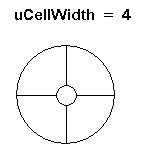PIX_RAD | PIX_WPER the size, in degrees of the cells around the CenterPt. That is, the circle around the CenterPt is divided into cells of uCellWidth degrees, as shown below: 1 to 360. The circle can be divided into cells of 1 degree to 360 degrees.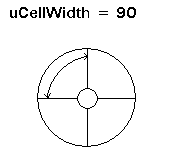PIX_RECT | PIX_WFRQ the number of cells present across the width of the bitmap 1 to the image width, if there is no region. If there is a region, then the range of values is just the region width.
PIX_RECT | PIX_WPER the width of each rectangular cell, in pixels 1 to the image width, if there is no region. If there is a region, then the range of values is just the region width.

### L_UINT uCellHeight

Value that represents the height of a rectangular cell, the number of rectangular cells present across the height of the bitmap, the number of cells along the radius of a circular cell, or the length of each cell along the radius of a circular cell, based on the flags set in `uFlags`.

If uFlags contains: then, uCellHeight contains: and the range of possible values for uCellHeight is:
PIX_RAD | PIX_HFRQ the number of cells present along the radius of the circular cell. 1 to the diagonal of the image, if there is no region. If there is a region, then the range of values is just the diagonal of the region rectangle.
PIX_RAD | PIX_HPER the radial length of each circular cell, in pixels 1 to the diagonal of the image, if there is no region. If there is a region, then the range of values is just the diagonal of the region rectangle.
PIX_RECT | PIX_HFRQ the number of cells present across the height of the bitmap 1 to the image height, if there is no region. If there is a region, then the range of values is just the region height.
PIX_RECT | PIX_HPER the height of each rectangular cell, in pixels 1 to the image height, if there is no region. If there is a region, then the range of values is just the region height.

### L_UINT uOpacity

Value that represents how transparent the cells are, compared to the original pixels. This is a percentage. Possible values are 0 100. (0 is the total transparency and 100 is the total opacity.)

### POINT CenterPt

Point that represents the center of revolution when circular cells are used. This parameter will be ignored if PIX_RECT is set in uFlags.

### L_UINT uFlags

Flags that indicate the values used to fill the cells, the shape of the cells, and what type of data is in the `uCellWidth` and `uCellHeight` parameters. You can use a bitwise OR (|) to specify one flag from each group.

#### The following are the flags indicate what values will be used to fill the cells:

Value Meaning
PIX_MAX [0x0000] Fill the cell with its maximum pixel value.
PIX_MIN [0x0001] Fill the cell with its minimum pixel value.
PIX_AVR [0x0002] Fill the cell with its average pixel value.

#### The following are the flags that indicate the shape of the cells:

Value Meaning
PIX_RECT [0x0000] Divide the image into rectangular cells. If this flag used, the uCellWidth value contains the cell width in pixels or the number of cells across the width of the bitmap. uCellHeight contains the cell height in pixels or the number of cells across the height of the bitmap.
PIX_RAD [0x0010] Divide the image into circular cells, centered around `CenterPt`. If this flag is used, the uCellWidth value determines the angular component of the cell, and uCellHeight determines the radial component of the cell. Please note that PIX_RAD must be OR-ed with PIX_WPER or PIX_WFRQ

#### The following are the flags the indicate what information is contained in the `uCellWidth` and `uCellHeight` parameters:

Value Meaning
PIX_WFRQ [0x0100] Indicates the number of cells along the width of the bitmap if rectangular cells are used. If circular cells are used, this indicates the number of cells present around the center of the circle.
PIX_WPER [0x0200] Indicates the width, in pixels of a cell, if rectangular cells are used. If circular cells are used, this indicates the number of degrees in each cell around the center point.
PIX_HFRQ [0x0400] Indicates the number of cells along the height of the bitmap if rectangular cells are used. If circular cells are used, this indicates the number of cells present along the radius of the circle.
PIX_HPER [0x0800] Indicates the height, in pixels of a cell, if rectangular cells are used. If circular cells are used, this indicates the radial length of each cell along the radius.

#### Returns

Value Meaning
SUCCESS The function was successful.
< 1 An error occurred. Refer to Return Codes.

This function does not support signed data images. It returns the error code ERROR_SIGNED_DATA_NOT_SUPPORTED if a signed data image is passed to this function.

This function will divide the image into rectangular or circular cells.

The uFlags parameter indicates whether to use rectangular or circular cells and indicates the type of information in the other parameters.

If the image is divided into circular cells by setting PIX_RAD in the `uFlags` parameter, the cells will be centered around the specified `CenterPt`. This center point must be defined inside the bitmap or inside the region, if the bitmap has a region. If the bitmap has a region, the effect will be applied on the region only.

This function supports 12 and 16-bit grayscale and 48 and 64-bit color images. Supportfor 12 and 16-bit grayscale and 48 and 64-bit color images is available in the Document and Medical Imaging toolkits.

To update a status bar or detect a user interrupt during execution of this function, refer to L_SetStatusCallback.

An example of circular cell division can be seen below:

This is the original image: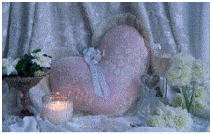The image below is the result of the following settings:

• uFlags = PIX_RAD | PIX_WPER | PIX_HPER | PIX_AVR
• uCellWidth = 90
• uCellHeight = 40

This indicates the circular cells are divided into 90 degree cell divisions and each cell has a radial length of 40 pixels. Each cell division is filled with the average value for that cell division.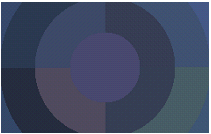The image below is the result of the following settings:

• uFlags = PIX_RAD | PIX_WFRQ | PIX_HFRQ | PIX_AVR
• uCellWidth = 90
• uCellHeight = 40

This indicates the circular cells are divided into 90 separate cell divisions around the center point and there are 40 cell divisions along the radius. Each cell division is filled with the average value for that cell division.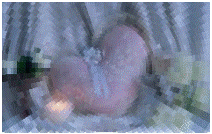This function does not support 32-bit grayscale images. It returns the error code ERROR_GRAY32_UNSUPPORTED if a 32-bit grayscale image is passed to this function.

Required DLLs and Libraries

Platforms

Win32, x64.

## Example

This example applies a pixelation effect to the bitmap.

````L_INT PixelateBitmapExample(L_VOID) `
`{ `
`   L_INT nRet; `
`   BITMAPHANDLE LeadBitmap;   /* Bitmap handle for the image */ `
`   POINT CenterPt;  `
` `
`   /* Load a bitmap at its own bits per pixel  */ `
`   nRet = L_LoadBitmap (MAKE_IMAGE_PATH(TEXT("sample5.cmp")), &LeadBitmap, sizeof(BITMAPHANDLE), 0, ORDER_BGR, NULL, NULL); `
`   if(nRet !=SUCCESS) `
`      return nRet; `
`   /* divide the image in to circular cells with angle length  = 5°, and radius = 10 */ `
`   CenterPt.x  =  LeadBitmap.Width/2; `
`   CenterPt.y  =  LeadBitmap. Height/2; `
`   nRet = L_PixelateBitmap (&LeadBitmap, 5, 10, 100, CenterPt, PIX_AVR | PIX_RAD | PIX_WPER | PIX_HPER); `
`   if(nRet !=SUCCESS) `
`      return nRet; `
`   nRet = L_SaveBitmap(MAKE_IMAGE_PATH(TEXT("Result.BMP")), &LeadBitmap, FILE_BMP, 24, 0, NULL); `
`   if(nRet !=SUCCESS) `
`      return nRet; `
`   //free bitmap  `
`   if(LeadBitmap.Flags.Allocated)   `
`      L_FreeBitmap(&LeadBitmap);   `
`   return SUCCESS; `
`} ````

Help Version 20.0.2019.12.5